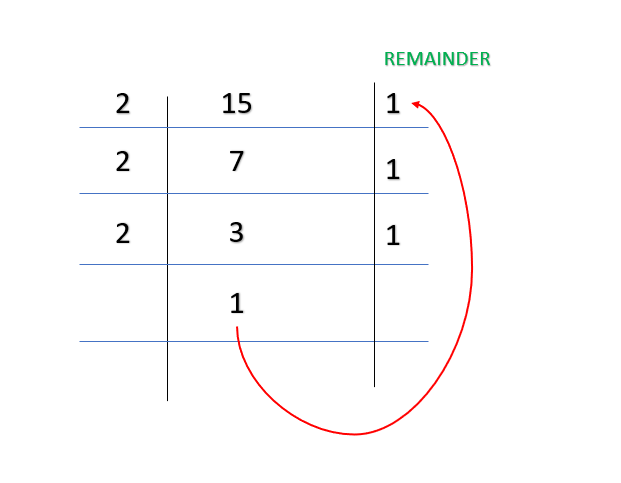# Python program to print the binary value of the numbers from 1 to N

• Last Updated : 24 Jan, 2021

Given a positive number N, the task here is to print the binary value of numbers from 1 to N. For this purpose various approaches can be used.

The binary representation of a number is its equivalent value using 1 and 0 only. Example for k = 15, binary value is 1 1 1 1WORKING FOR METHOD 1

### Method 1: Using the Elementary method with recursion.

Approach

• Divide k by 2.
• Recursive call on the function and print remainder while returning from the recursive call.
• Repeat the above steps till the k is greater than 1.
• Repeat the above steps till we reach N

Program:

## Python3

 `# code to print binary values of first 5 numbers`` ` `# recursive function``def` `Print_Binary_Values(num):``    ``# base condition``    ``if``(num > ``1``):``        ``Print_Binary_Values(num ``/``/` `2``)``    ``print``(num ``%` `2``, end``=``"")`` ` ` ` `# driver code``if` `__name__ ``=``=` `"__main__"``:``    ``N ``=` `5`` ` `    ``# looping N times``    ``for` `i ``in` `range``(``1``, N``+``1``):``        ``Print_Binary_Values(i)``        ``print``(end``=``" "``)`

Output

1 10 11 100 101

### Method 2: Using Bitwise Operator.

Approach

• Check if k > 1
• Right shift the number by 1 bit and perform a recursive call on the function
• Print the bits of number
• Repeat the steps till we reach N

Program:

## Python3

 `# code to print binary values of first 5 numbers`` ` `# recursive function`` ` ` ` `def` `Print_Binary_Values(num):`` ` `    ``# base condition``    ``if``(num > ``1``):``        ``Print_Binary_Values(num >> ``1``)``    ``print``(num & ``1``, end``=``"")`` ` ` ` `# driver code``if` `__name__ ``=``=` `"__main__"``:``    ``N ``=` `5`` ` `    ``# looping N times``    ``for` `i ``in` `range``(``1``, N``+``1``):``        ``Print_Binary_Values(i)``        ``print``(end``=``" "``)`

Output

1 10 11 100 101

### Method 3: Using Inbuilt Library of Python

bin() is an inbuilt python function that can convert any decimal number given to it as input to its equivalent binary.

Syntax:

bin (number)

here number is the decimal number that gets converted to binary

Program

## Python3

 `# code to print first 5 binary number using builtIn library`` ` ` ` `def` `Print_Binary_Number(num):``    ``for` `i ``in` `range``(``1``, num``+``1``):`` ` `        ``# using bin to print binary value``        ``print``(``int``(``bin``(i).split(``'0b'``)[``1``]), end``=``" "``)`` ` ` ` `# driver code``if` `__name__ ``=``=` `"__main__"``:``    ``num ``=` `5``    ``Print_Binary_Number(num)`

Output

1 10 11 100 101

My Personal Notes arrow_drop_up